Courses

# Electrochemistry

## 20 Questions MCQ Test GATE Chemistry Mock Test Series | Electrochemistry

Description
This mock test of Electrochemistry for Chemistry helps you for every Chemistry entrance exam. This contains 20 Multiple Choice Questions for Chemistry Electrochemistry (mcq) to study with solutions a complete question bank. The solved questions answers in this Electrochemistry quiz give you a good mix of easy questions and tough questions. Chemistry students definitely take this Electrochemistry exercise for a better result in the exam. You can find other Electrochemistry extra questions, long questions & short questions for Chemistry on EduRev as well by searching above.
QUESTION: 1

### For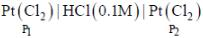cell reaction will be spontaneous if:

Solution: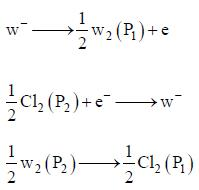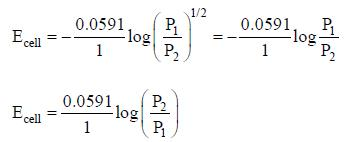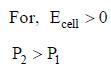QUESTION: 2

### Equivalent conductance of saturated BaSO4 is 400 ohm-1cm2 equivalemt-1 and specific conductance is 8 x 10-5 ohm-1cm-1. Hence, ksp of BaSO4 is:

Solution: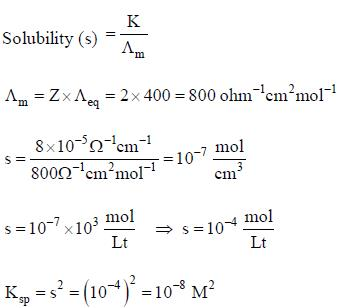*Answer can only contain numeric values
QUESTION: 3

### For a cell reaction, E (at 25oC) = 1.26V, n = 2 and ΔS = -96.5JK-1mol-1. E at 85oC by assuming ΔS to be independent of temperature is _____ (V). [Given F = 96500 Cmol-1] (Round off to two decimal places).

Solution: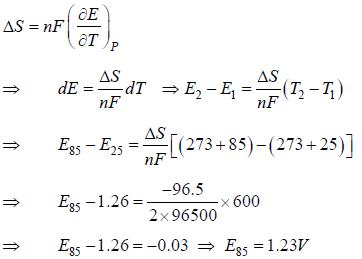*Answer can only contain numeric values
QUESTION: 4

Given for the reaciton,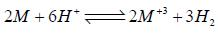for which ΔHo = -3.0 kcal. The molar entropies are 6.5 cal/K for M, -22.2 cal/K for M+3, 31.2 cal/k for H2 and -10.0cal/K for H+.
The value of Eo for the half cell,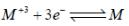is ______[Round off to two decimal places]

Solution: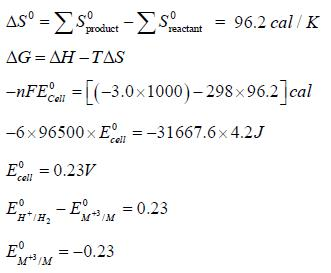QUESTION: 5

The resistance of decinormal solution of a salt occupying a volume between two platinum electrodes 1.80 cm apart and 5.4 cm2 area was found to be 32 ohm. The value of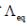is

Solution: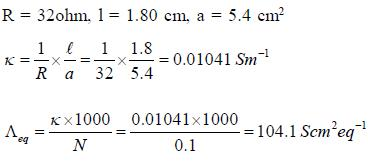QUESTION: 6

The value of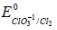Given :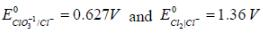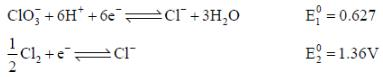The required electrode reaction is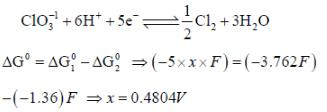Solution: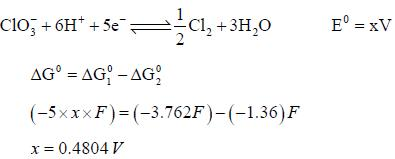QUESTION: 7

A current of 0.5A is passed through molten AlCl3 for 40 min. The mass of the aluminum deposited at the cathode is (Al = 27)

Solution: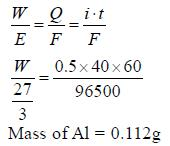QUESTION: 8

At 180C the mobilities of NH+4 and ClO-4 ions are 6.6 x 10-4 and 5.7 x 10-4 cm2 volt-1s-1 at infinite dilution. The value of equivalent conductance of ammonium perchlorate solution at infinite dilution

Solution: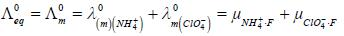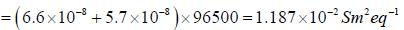*Answer can only contain numeric values
QUESTION: 9

For the reaction,
H2(g)(1atm) + 2AgCL(s) = 2Ag(s) + 2H+(aq)(0.1M)+2Cl-(aq)(0.1M)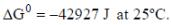The emf of the cell is _____volt.(Round off to two decimal placs)

Solution: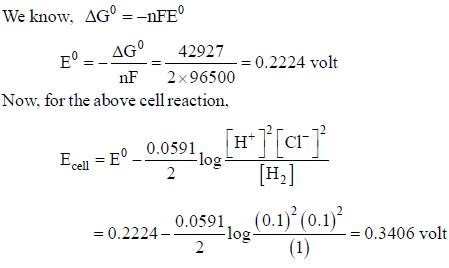QUESTION: 10

The variation of equivalent conductance of strong electrolyte with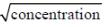is correctly shown in the figure

Solution:

With dilution λeq increases in a straight line for strong electrolyte.

QUESTION: 11

According to Debye Huckel limiting law the mean activity coefficient of 0.5 x 10-4 mol / kg CaCl2 is at 25oC

Solution: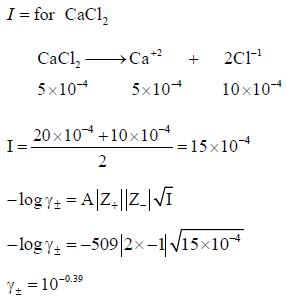*Answer can only contain numeric values
QUESTION: 12

Given that, E0(Fe3+,Fe) = -0.04V and E0(Fe2+,Fe) = -0.44V, the value of E0(Fe3+,Fe2+) is _______ (in V). (round off to two decimal places).

Solution: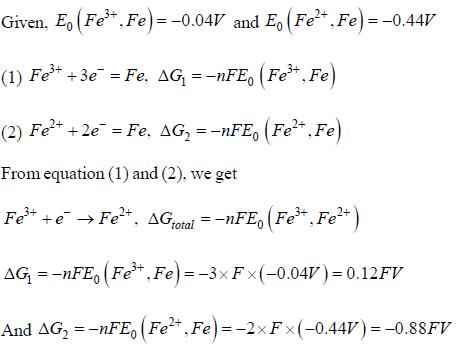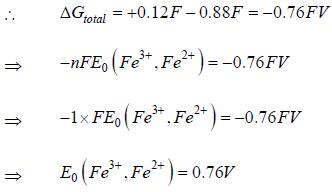*Answer can only contain numeric values
QUESTION: 13

The solubility product of AgBr(s) is 5 x 10-13 at 298k. If the standard reduction potential of the half cell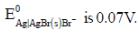The standard reduction potential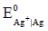(in volts) is _____ (round off to two decimal places).

Solution: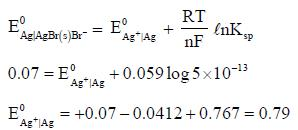*Answer can only contain numeric values
QUESTION: 14

The concentration of a MgSO4 solution having the same ionic strength as that of a 0.1M Na2SO4 solution is ____(Round off to three decimal places)

Solution: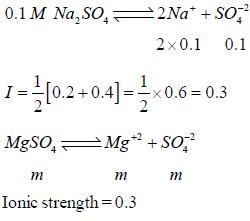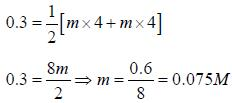QUESTION: 15

The temperature coefficient of the cell is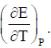Choose the incorrect statement(S)

Solution: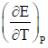= 0 then |ΔH| > |nFE| endothermic reaction (Wrong relation)

QUESTION: 16

The standard electrode potential Eo at a fixed temperature and in a given medium is dependent on

Solution:

Depend only electrode reaction and electrode composition

QUESTION: 17

At 273K, the calorimetric determination of ΔH for the reaction,
Zn(s) + 2AgCl(s) → ZnCl2(aq) + Ag(s)
yielded -217.78 kJ mol-1, while the emf of the corresponding cell was 1.015 V. The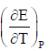of the cell is

Solution: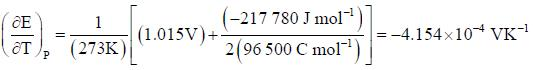QUESTION: 18

The Zn2+|Zn half cell (Eθ = -0.762V) is connected to a Cu2+ | Cu halff cell (Eo = 0.340V). The value of log10K, where K is the equilibrium constant is [Given : (2.303 RT/F) = 0.06]

Solution: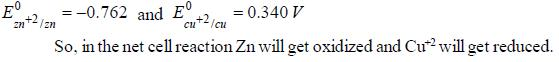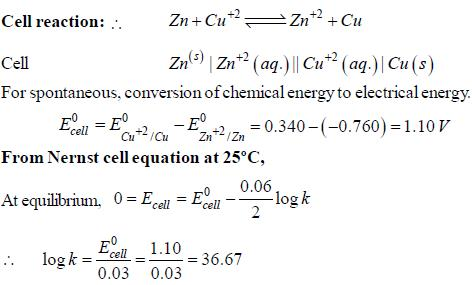QUESTION: 19

An electrochemical cell consists of two half-cell reactions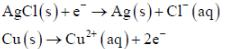The mass of copper (in grams) dissolved on passing 0.5 A current for 1 hour is
[Given: atoms mass of Cu is 63.6; F = 96500C mol-1]

Solution: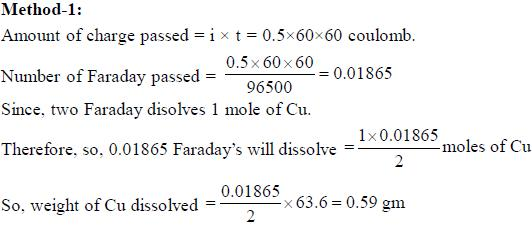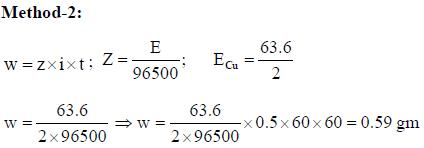QUESTION: 20

According to the Dubye-Huckel limiting law, if the concendration of the dilute aqueous solution of KCI is increased 4-fold, the value of log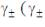is the molal mean ionic activity coefficient) will be

Solution: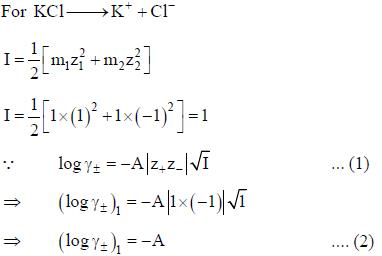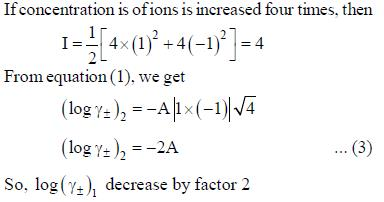Track your progress, build streaks, highlight & save important lessons and more!

### Similar Content### Related tests